Question

# Question 1 1 pts Find the derivative of f(x) = cos(sin(3x)). Of"(x) --cos(3x) sin(sin(3x)) O f'()...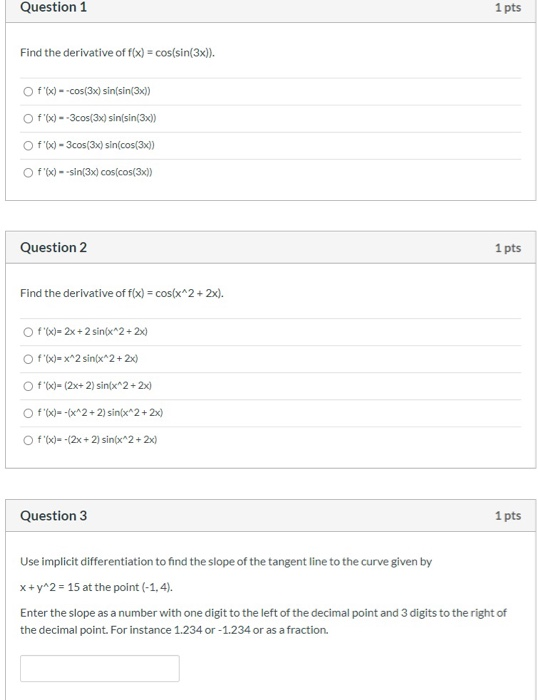Question 1 1 pts Find the derivative of f(x) = cos(sin(3x)). Of"(x) --cos(3x) sin(sin(3x)) O f'() -- 3cos(3x) sin(sin(3x)) Of'(x) - 3cos(3x) sin(cos(3x]) f'x) --sin(3x) cos(cos(3x)) Question 2 1 pts Find the derivative of f(x) = cos(x^2 + 2x). Of "(x)=2x+2 sin(x^2 + 2x) O f'(x)= x^2 sin(x^2+2x) Of"(x)= (2x+ 2) sin(x^2 + 2x) f'(x)= -(x^2 + 2) sin(x^2 + 2x) O f'(x)--(2x + 2) sin(x^2 + 2x) Question 3 1 pts Use implicit differentiation to find the slope of the tangent line to the curve given by X+ y^2 = 15 at the point (-1,4). Enter the slope as a number with one digit to the left of the decimal point and 3 digits to the right of the decimal point. For instance 1.234 or -1.234 or as a fraction.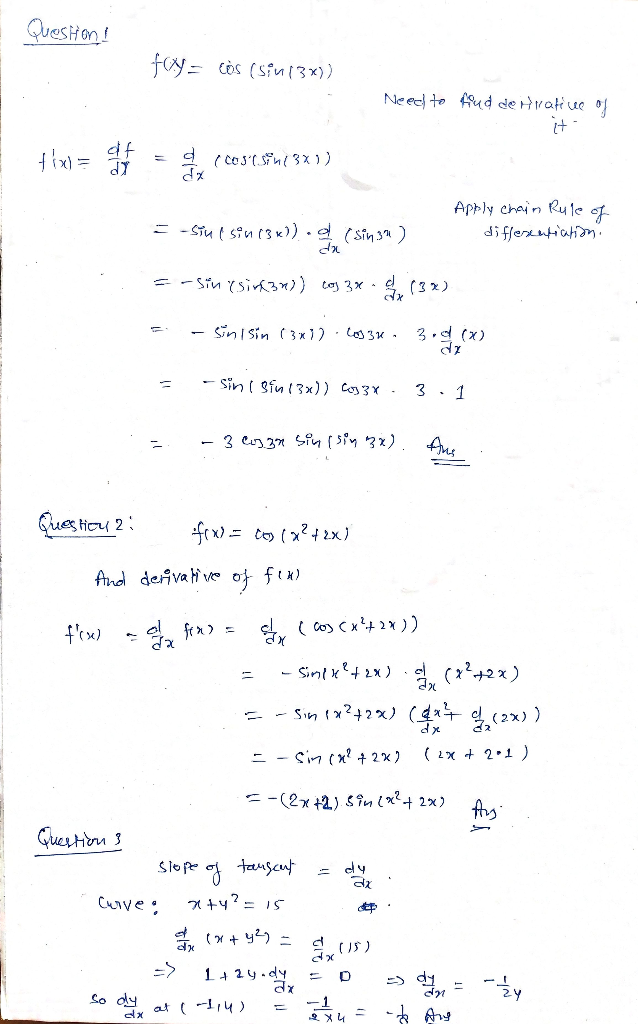Though we can answer one question at time but we have provided solutions to all 3 question.

#### Earn Coins

Coins can be redeemed for fabulous gifts.

Similar Homework Help Questions
• ### 1. Express the limit as a derivative and evaluate. 17 lim 16+h-2 lim 2. Calculate y. tan x 1 + co...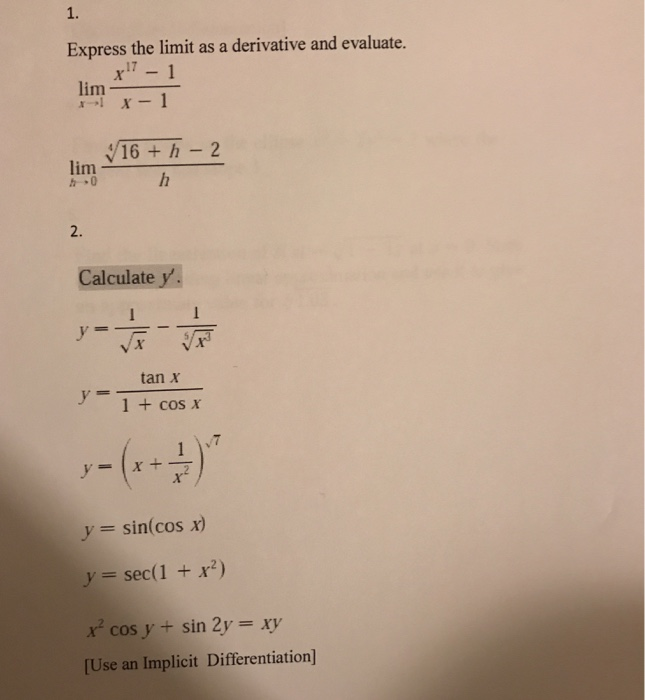1. Express the limit as a derivative and evaluate. 17 lim 16+h-2 lim 2. Calculate y. tan x 1 + cos x y sin(cos x) y= sec(1 +x2) x cos y + sin 2y xy Use an Implicit Differentiation] 3. Find y" if x, y,6-1. [Use Implicit Differentiation] 4. Find an equation of the tangent to the curve at the given point. 121 12+ 1 [Use Implicit Differentiation] 4. Find the points on the ellipse x2 + tangent line has...

• ### # 2,3,4,7, 10,11,15,18) Differentiate the function: #2 f(x) = ln(22 + 1) #3 f@) = ln(cos)...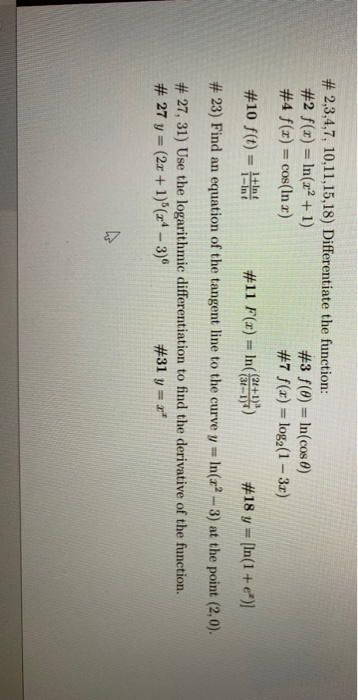# 2,3,4,7, 10,11,15,18) Differentiate the function: #2 f(x) = ln(22 + 1) #3 f@) = ln(cos) #4 f(x) = cos(In x) #7 f(x) = log2(1 – 3x) #10 f(t) = 1+Int #11 F(x) = In( 3+1") #18 y = (ln(1 + e*)] # 23) Find an equation of the tangent line to the curve y = In(x2 – 3) at the point (2,0). # 27, 31) Use the logarithmic differentiation to find the derivative of the function. # 27 y...

• ### Question 2-Part B: How many inflection points for the function whose second derivative is f"(x) sin(3x)-cos(x2)...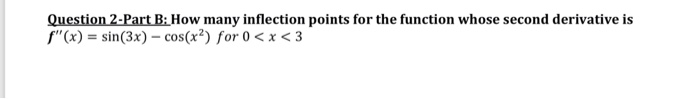Question 2-Part B: How many inflection points for the function whose second derivative is f"(x) sin(3x)-cos(x2) for 0 < x < 3 Question 2-Part B: How many inflection points for the function whose second derivative is f"(x) sin(3x)-cos(x2) for 0

• ### Let g(x) = sin (cos x^3) Find g ' (x): The choices are a) -3x^2sinx^3cos(cos x^3) b) -3x^2sinx^3sin(cos x^3) c) -3x^2cosx^3sin(cos x^3) d) 3x^2sin^2(cos x^3) I'm not exactly sure where I should start

Let g(x) = sin (cos x^3) Find g ' (x):The choices are a) -3x^2sinx^3cos(cos x^3)b) -3x^2sinx^3sin(cos x^3)c) -3x^2cosx^3sin(cos x^3)d) 3x^2sin^2(cos x^3)I'm not exactly sure where I should start.Should I begin with d/dx of sin? Or do the inside derivative first...and do I have to separate cos and x^3 as well?

• ### D1.1. Evaluate f'(a) by using the definition of derivative of a function f(x) = 4x2 +...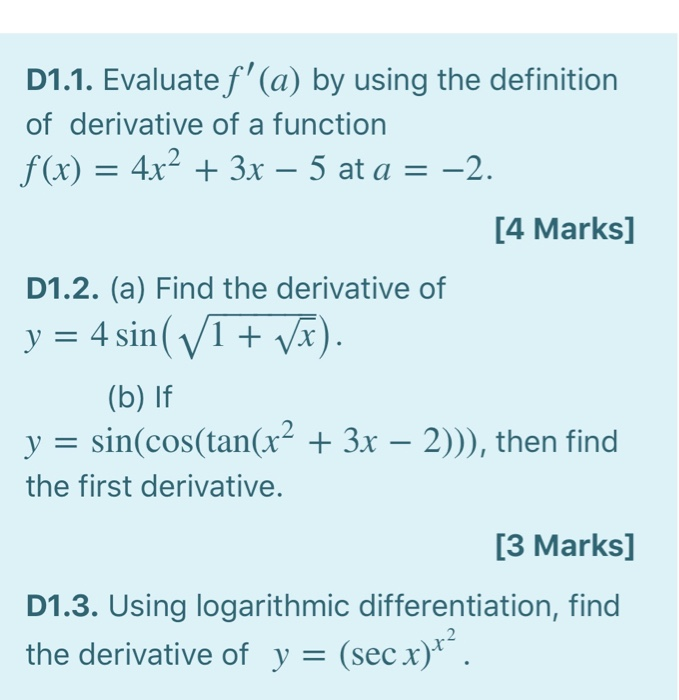D1.1. Evaluate f'(a) by using the definition of derivative of a function f(x) = 4x2 + 3x – 5 at a = -2. [4 Marks] D1.2. (a) Find the derivative of y = 4 sin( V1 + Vx). (b) If y = sin(cos(tan(x2 + 3x – 2))), then find the first derivative. [3 Marks] D1.3. Using logarithmic differentiation, find the derivative of y = (sec x)+”.

• ### 9 - sin (1 point) Find the derivative of f(x) = 5 - COS f'(x) =...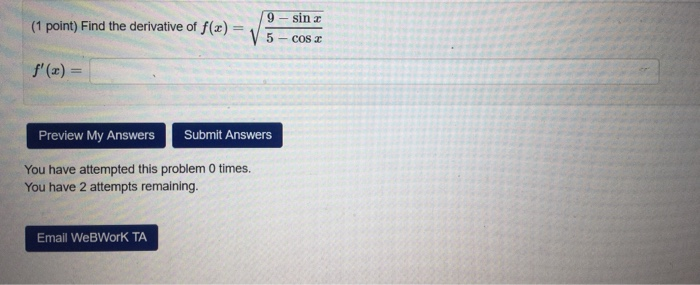9 - sin (1 point) Find the derivative of f(x) = 5 - COS f'(x) = Preview My Answers Submit Answers You have attempted this problem 0 times. You have 2 attempts remaining. Email WebWork TA

• ### Question Below are the graphs of f(x)= x²-3x²+1 and x² + y² = 2x²y2 a) Find...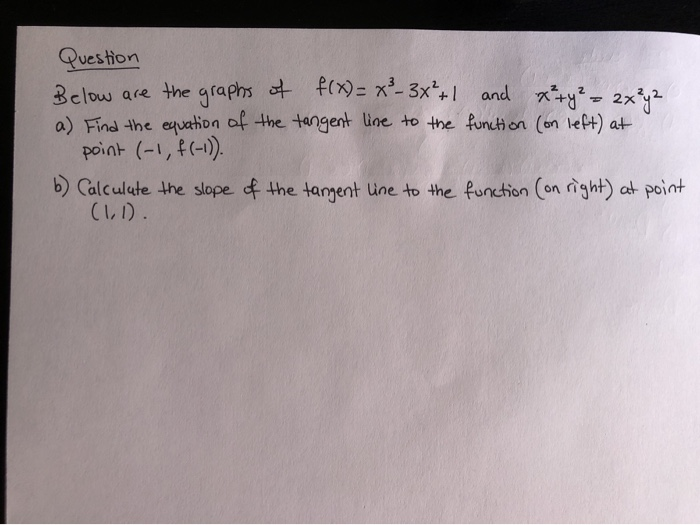Question Below are the graphs of f(x)= x²-3x²+1 and x² + y² = 2x²y2 a) Find the equation of the tangent line to the function (on left) at point (-1, f(-1)). 6) Calculate the slope of the tangent line to the function (on right) at point (1,1). 1- . 0,5 1 1.5 1 ST -1,8-1,694 -12 -1 -6.8-06-11-02 BIG 1 112 1,6 10

• ### QA: Pick two of the following functions: sin(3x)*sin(2x) cos(3x)*sin(2x) cos(3x)*sin(1x) cos(3x)*cos(2x) Find the integral of those...

QA: Pick two of the following functions: sin(3x)*sin(2x) cos(3x)*sin(2x) cos(3x)*sin(1x) cos(3x)*cos(2x) Find the integral of those functions from x= -pi to pi Show all work. hint : graph & use symmetry.

• ### Question 15 4 pts Use implicit differentiation to find the specified derivative at the given point....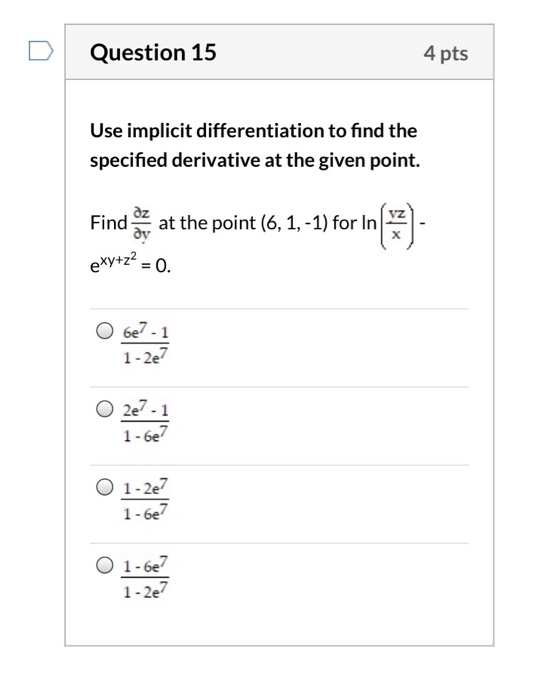Question 15 4 pts Use implicit differentiation to find the specified derivative at the given point. az Find at the point (6, 1,-1) for In exy+z2 = 0 6e7-1 1-2e 2e7- 1-6e O 1-2e 1-6e7 1-6e7 1-2e7 Question 15 4 pts Use implicit differentiation to find the specified derivative at the given point. az Find at the point (6, 1,-1) for In exy+z2 = 0 6e7-1 1-2e 2e7- 1-6e O 1-2e 1-6e7 1-6e7 1-2e7

• ### a) b) 54-1+1 Use logarithmic differentiation to find the first derivative of y= (3x² +2) 13...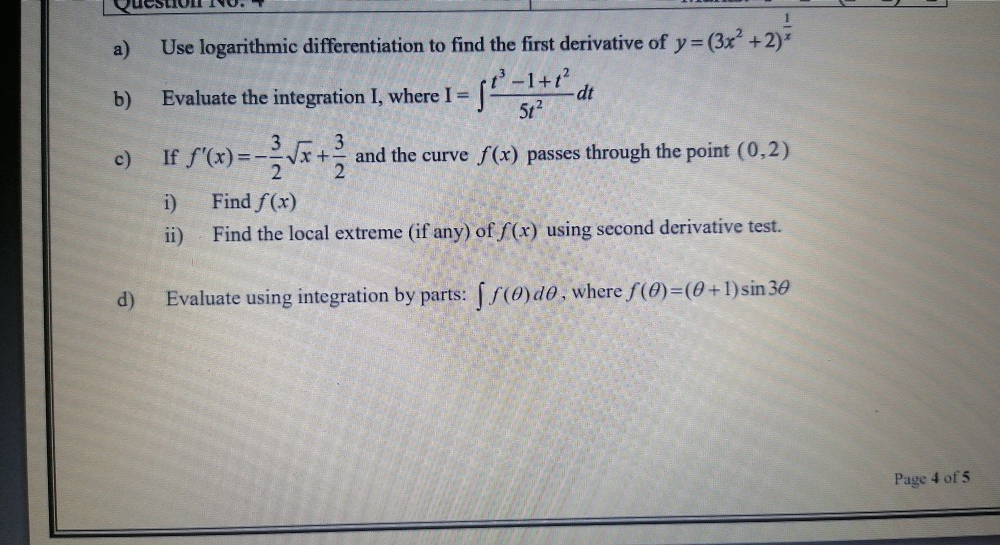a) b) 54-1+1 Use logarithmic differentiation to find the first derivative of y= (3x² +2) 13 Evaluate the integration I, where I = dt 5t2 3 If f'(x) == Vx+ and the curve f(x) passes through the point (0,2) 2 2 i) Find f(x) 11) Find the local extreme (if any) of f(x) using second derivative test. d) Evaluate using integration by parts: 5 (0)do, where f(O)=(0+1) sin 30 Page 4 of 5